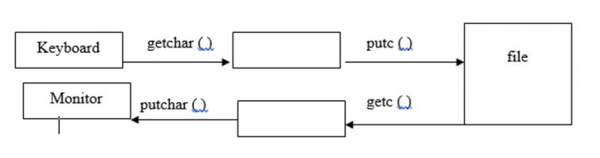# Explain putc() and getc() functions of files in C language

File is collection of records or is a place on hard disk, where data is stored permanently.

## Operations on files

The operations on files in C programming language are as follows −

• Naming the file
• Opening the file
• Writing into the file
• Closing the file

## syntax

The syntax for opening a file is as follows −

FILE *File pointer;

For example, FILE * fptr;

The syntax for naming a file is as follows −

File pointer = fopen ("File name", "mode");

For example,

fptr = fopen ("sample.txt", "r");
FILE *fp;
fp = fopen ("sample.txt", "w");

## putc( ) and getc( ) functions

putc( ) function is used for writing a character into a file.

The syntax for putc() function is as follows −

putc (char ch, FILE *fp);

For example,

FILE *fp;
char ch;
putc(ch, fp);

getc( ) function is used to read a character from file.

The syntax for getc() function is as follows −

char getc (FILE *fp);

For example,

FILE *fp;
char ch;
ch = getc(fp);## Example

Following is the C program for using putc() and getc() functions −

Live Demo

#include<stdio.h>
int main(){
char ch;
FILE *fp;
fp=fopen("std1.txt","w"); //opening file in write mode
printf("enter the text.press cntrl Z:");
while((ch = getchar())!=EOF){
putc(ch,fp); // writing each character into the file
}
fclose(fp);
fp=fopen("std1.txt","r");
printf("text on the file:");
while ((ch=getc(fp))!=EOF){ // reading each character from file
putchar(ch); // displaying each character on to the screen
}
fclose(fp);
return 0;
}

## Output

When the above program is executed, it produces the following result −

enter the text.press cntrl Z:
Hi Welcome to TutorialsPoint
Here I am Presenting Question and answers in C Programming Language
^Z
text on the file:
Hi Welcome to TutorialsPoint
Here I am Presenting Question and answers in C Programming Language